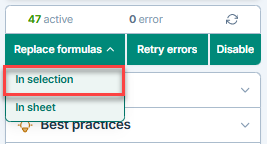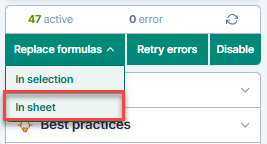# Replace GPT formulas with their results

Convert GPT formulas to static values and prevent any further recalculation, either on a selected number of cells or across an entire sheet. If you need to recover the original formulas later, you can use the version history of your Google spreadsheet.

Prerequisites
• You have opened a spreadsheet containing GPT formulas.
• You have opened the GPT for Sheets and Docs add-on by clicking Extensions > GPT for Sheets and Docs > Open.

## Replace formulas in selection​

1. Select the cell(s) which contain formulas you want to replace.

2. Open the GPT formulas controls dropdown.

3. Click In selection in the Replace GPT formulas with results section.Warning

Always select the full range of results for formulas like GPT_LIST to prevent data loss.

GPT formulas within the selected cells are replaced by their respective results.

## Replace formulas in current sheet​

1. Open the GPT formulas controls dropdown.

2. Click In sheet under the Replace GPT formulas with results section.All GPT formulas within the current sheet are replaced by their corresponding results.

## Formula replacement limit​

Working with selections larger than 10,000 cells may negatively impact performance. For best results, keep the number of GPT formulas on one sheet under 10,000.